# Pandas Introduction¶

## Part II¶

If you're continuing using the same notebook as in part one, you won't need to import anything extra. If this is a new notebook, you'll need to import the numpy and pandas libraries as well as the Series and DF objects from the pandas library like so.

In :
import numpy as np
import pandas as pd
import seaborn as sb

from numpy.random import randn
import matplotlib.pyplot as plt
from matplotlib import rcParams
from pandas import Series, DataFrame


In :
%matplotlib inline

# Figsize is represented in inches.
rcParams['figure.figsize']= 5,4
sb.set_style('whitegrid')


In the previous demonstration, we covered some of the basics of with dataframe objects, basic data munging, and some visualization. In part two, we'll start to try to start using the data and generating new and usefull values from what we have.

## Profit Calculator¶

This takes two dataframes and concatinates them based on a common key. This is handy if you have sales data on a platform like Google Analytics while keeping the details of your profit margins offline. In this way you can merge them and do your own offline analytics while keeping the details of your specific margins private.

This would then make it easy to maintain a single list of your products and bump it against a sales report from any time period. Below we read in the test analytics data. Set the sku data type to string, and specify which columns to use.

In :
Sales = pd.read_csv("./Test.csv", parse_dates=True, dtype = {"sku":str}, usecols = ["date", "price", "sku"])
Sales

Out:
date price sku
0 22 Aug 2013 72 445
1 22 Aug 2013 56 203
2 22 Aug 2013 56 203
3 22 Aug 2013 115 661
4 04 Sep 2013 115 661
5 11 Sep 2013 72 445
6 28 Sep 2013 72 445
7 28 Sep 2013 117 661
8 02 Oct 2013 72 445
9 02 Oct 2013 56 203
10 02 Oct 2013 115 661

Here we create our product database which maps a product sku with its cost. I added some spacing in the cost column to make it easier to see which product maps to which SKU, but that's not necessary.

In :
# Cost of goods sold COGS
COGS = DataFrame({'sku':['203','445', '661'],
'cost':[  46,   53,   89]})

COGS

Out:
cost sku
0 46 203
1 53 445
2 89 661

Once we have our two dataframes, we merge them by calling the merge method and passing in our two dataframes. We merge using "left" or the AD DataFrame as the primary source and we look for matches "on" the SKU column. This produces a new DF that contains all of the data in one spot.

In :
# Create a new frame by joining the original two, merging by matching sku numbers to the main DF
C = pd.merge(Sales, COGS, how = 'left', on='sku')
print(C)

           date  price  sku  cost
0   22 Aug 2013     72  445    53
1   22 Aug 2013     56  203    46
2   22 Aug 2013     56  203    46
3   22 Aug 2013    115  661    89
4   04 Sep 2013    115  661    89
5   11 Sep 2013     72  445    53
6   28 Sep 2013     72  445    53
7   28 Sep 2013    117  661    89
8   02 Oct 2013     72  445    53
9   02 Oct 2013     56  203    46
10  02 Oct 2013    115  661    89


If you want to change the order of the columns to keep certain data next to each other, you can simply set the order in a list and pass it in as a parameter to the DF.

In :
Col_Order = ['date', 'sku','cost', 'price']
C1 = DataFrame(C, columns=Col_Order)

C1

Out:
date sku cost price
0 22 Aug 2013 445 53 72
1 22 Aug 2013 203 46 56
2 22 Aug 2013 203 46 56
3 22 Aug 2013 661 89 115
4 04 Sep 2013 661 89 115
5 11 Sep 2013 445 53 72
6 28 Sep 2013 445 53 72
7 28 Sep 2013 661 89 117
8 02 Oct 2013 445 53 72
9 02 Oct 2013 203 46 56
10 02 Oct 2013 661 89 115

So now that we have the cost and the price listed in one DF, how do we calculate the profit and start making use of this data? Simple, just call the DF using the column indexes and set up an equasion like you might if you were working it out on paper.

In :
# Creates a profit series and calculates the difference between the
# price and the cost.
C['profit']=C['price']-C['cost']

# Sets the column order including new column.
cols = ['date', 'sku', 'cost', 'price', 'profit']

# Creates a new DF and passes in the source DF and desired column order.
Profit = DataFrame(C, columns=cols)
Profit

Out:
date sku cost price profit
0 22 Aug 2013 445 53 72 19
1 22 Aug 2013 203 46 56 10
2 22 Aug 2013 203 46 56 10
3 22 Aug 2013 661 89 115 26
4 04 Sep 2013 661 89 115 26
5 11 Sep 2013 445 53 72 19
6 28 Sep 2013 445 53 72 19
7 28 Sep 2013 661 89 117 28
8 02 Oct 2013 445 53 72 19
9 02 Oct 2013 203 46 56 10
10 02 Oct 2013 661 89 115 26

Now we can take the combined data and start looking a little deeper. Here I want to find what it costs me to do business every day, how much I charged, and how much profit I made. You can do that by grouping your data by a column, in this case the date, and using the sum() method.

In :
sales_by_date = Profit.groupby(Profit['date'])
sales_by_date.sum()

Out:
cost price profit
date
02 Oct 2013 188 243 55
04 Sep 2013 89 115 26
11 Sep 2013 53 72 19
22 Aug 2013 234 299 65
28 Sep 2013 142 189 47

Now you may be interested in visualizing that as a line graph. Now it's a littler easier to see your bottom line. Here you can see the relationship between the price of your product, the cost of goods, and the difference represented as profit on the bottom.

In :
sales_by_date.sum().plot()

Out:
<matplotlib.axes._subplots.AxesSubplot at 0x116b239b0>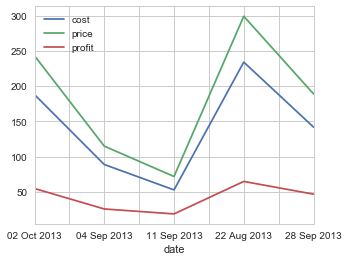You can break it down even further if you're only interested in your profit you can simply use the DF group and sum the profit series and plot that.

In :
sales_by_date['profit'].sum().plot()

Out:
<matplotlib.axes._subplots.AxesSubplot at 0x116ab3e48>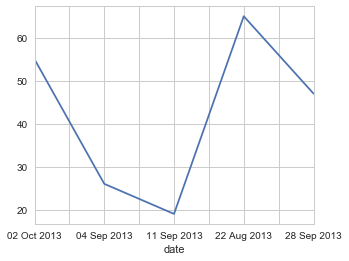Here we can group the our sales by item numbers and find the total cost of goods by item and associated profit.

In :
sales_by_date = Profit.groupby(Profit['sku'])
sales_by_date.sum()

Out:
cost price profit
sku
203 138 168 30
445 212 288 76
661 356 462 106

You could then display the visualization of your costs and profit by item type. Here we can see where our margins are tightest and where we can focus our marketing efforts. The return on item 445 is pretty close to that of 661; however, the cost per item is much lower. Perhaps it would make more sense to focuse on selling more 445. I could keep my overhead down, keep more in stock, and still do really well.

In :
sales_by_date.sum().plot(kind='bar')

Out:
<matplotlib.axes._subplots.AxesSubplot at 0x116cbae80>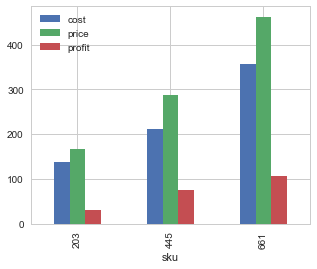## Vehicle Comparison¶

Here we take a closer look at our vehicle data again.

In :
# Reading in the data

# Setting the columns that you want to use.
# NOTE: You can chose the display order by reordering the columns the way you want.
cars.columns = ['car_names','mpg','cyl','disp', 'hp', 'drat', 'wt', 'qsec', 'vs', 'am', 'gear', 'carb']
cars.index = cars.car_names
# Returns the first five rows.

Out:
car_names mpg cyl disp hp drat wt qsec vs am gear carb
car_names
Mazda RX4 Mazda RX4 21.0 6 160.0 110 3.90 2.620 16.46 0 1 4 4
Mazda RX4 Wag Mazda RX4 Wag 21.0 6 160.0 110 3.90 2.875 17.02 0 1 4 4
Datsun 710 Datsun 710 22.8 4 108.0 93 3.85 2.320 18.61 1 1 4 1
Hornet 4 Drive Hornet 4 Drive 21.4 6 258.0 110 3.08 3.215 19.44 1 0 3 1
Hornet Sportabout Hornet Sportabout 18.7 8 360.0 175 3.15 3.440 17.02 0 0 3 2

Here we plot the efficiency of vehicles. On the x-axis we have the list of our vehciles. On the y-axis we display where they fall in respect to their miles per gallon.

In :
mpg = cars['mpg']

fig = plt.figure()
mpg.plot()

ax.set_xticks(range(32))

ax.set_xticklabels(cars.car_names, rotation=60, fontsize='medium')
ax.set_title('Miles per Gallon of Cars in mtcars')

ax.set_xlabel('car names')
ax.set_ylabel('miles/gal')

ax.legend(loc='best')

Out:
<matplotlib.legend.Legend at 0x112fd1240>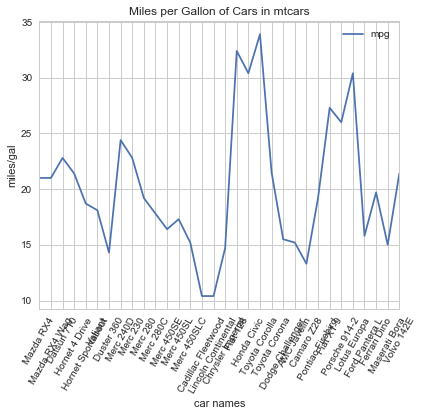You can also grab quick details about the dataset like:

In :
# Maximum value
mpg.max()

Out:
33.899999999999999
In :
# Minimum value
mpg.min()

Out:
10.4
In :
# Record count
mpg.count()

Out:
32

Or you could use the describe method to give a quick all-in-one summary. Describe generates descriptive statistics that summarize the central tendency, dispersion and shape of a dataset’s distribution, excluding NaN values.

In :
mpg.describe()

Out:
count    32.000000
mean     20.090625
std       6.026948
min      10.400000
25%      15.425000
50%      19.200000
75%      22.800000
max      33.900000
Name: mpg, dtype: float64

Therer are many useful ways to visualize your data to see relationships between data. Here we have a histogram plot that shows you the general distribution of the data.

In :
mpg.plot(kind='hist')

Out:
<matplotlib.axes._subplots.AxesSubplot at 0x112f2a828>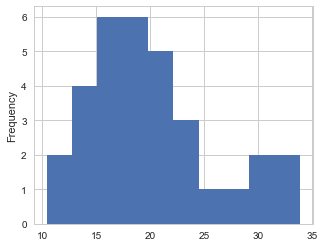You can also use seaborn to plot a histogram with distribution curve. This can be helfpul when determining if your data meets prerequisites for certain analytics techniques.

In :
sb.distplot(mpg)

Out:
<matplotlib.axes._subplots.AxesSubplot at 0x113144f60>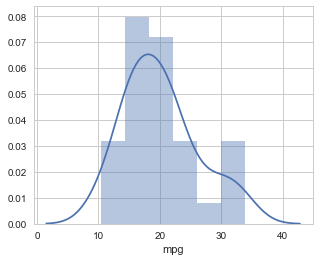Here we create a scatterplot from the two column series, "hp" and "mpg". The "s" attribute sets the dot size.

In :
cars.plot(kind='scatter', x='hp', y='mpg', c=['lightblue'], s=50)

Out:
<matplotlib.axes._subplots.AxesSubplot at 0x11701c908>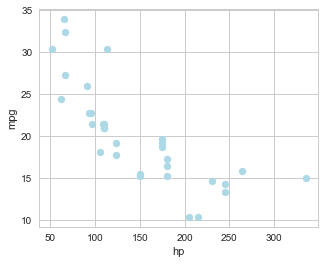Here we have the same scatterplot with trendline and confidence interval region. Here the interval was just randomly selected for demonstration purposes, not calculated.

In :
sb.regplot(x='hp', y='mpg', data=cars, scatter=True, ci=80)

Out:
<matplotlib.axes._subplots.AxesSubplot at 0x1176869e8>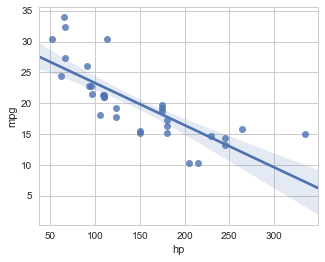Here you can create a grid matrix which evaluates the relationship between all the listed variables. Here we can see the relationship between automatic and manual cars with MPG, displacement, HP, and weight.

In :
cars_df = pd.DataFrame((cars.ix[:,(1,3,4,6)].values), columns = ['mpg', 'disp', 'hp', 'wt'])
cars_target = cars.ix[:,9].values
target_names = [0,1]

cars_df['group'] = pd.Series(cars_target, dtype="category")
sb.pairplot(cars_df, hue='group', palette='hls')

Out:
<seaborn.axisgrid.PairGrid at 0x1186f7ba8>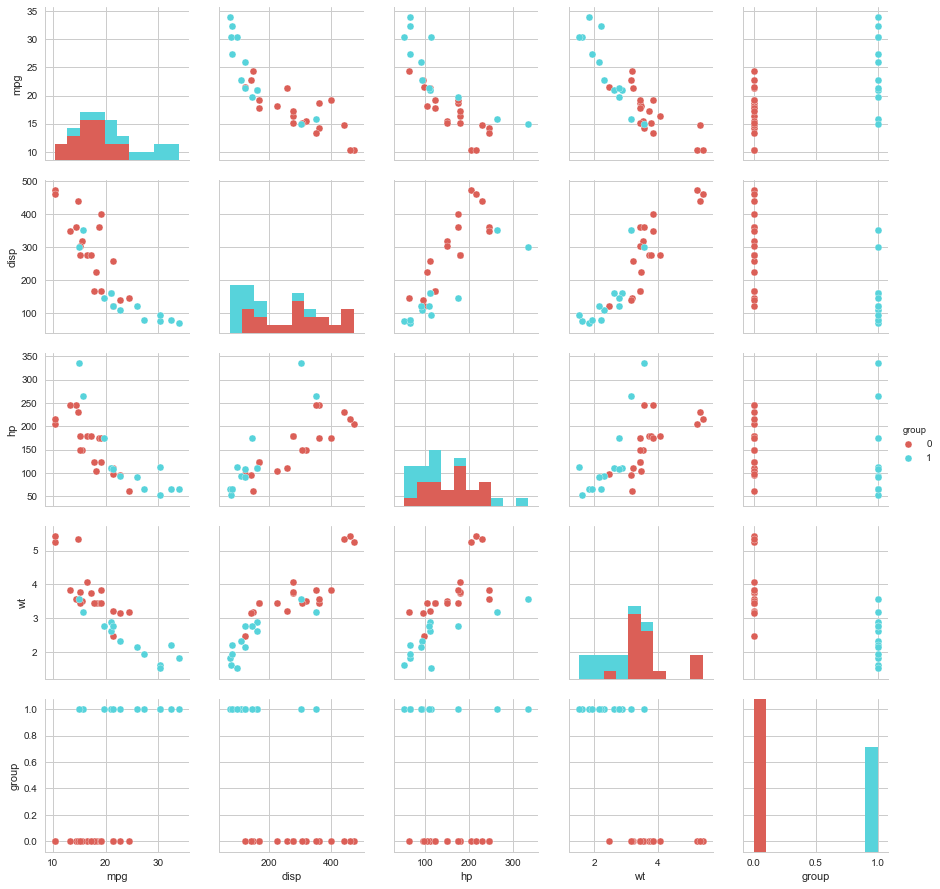## Math and Statistics with Pandas¶

You can set your desired precision by using the set_printoptions method with the precision attribute.

In :
np.set_printoptions(precision=2)


## Creating Arrays¶

You can create arrays by passing lists to the array method.

In :
a = np.array([1,2,3,4,5,6])
a

Out:
array([1, 2, 3, 4, 5, 6])

You can create a multidimensional array by passing in a list of lists.

In :
b = np.array([[10,20,30], [40,50,60]])
b

Out:
array([[10, 20, 30],
[40, 50, 60]])

Creating an array by assignment

In :
np.random.seed(25)
c = 36*np.random.randn(6)
c

Out:
array([  8.22,  36.97, -30.23, -21.28, -34.45,  -8.  ])
In :
d = np.arange(1,35)
d

Out:
array([ 1,  2,  3,  4,  5,  6,  7,  8,  9, 10, 11, 12, 13, 14, 15, 16, 17,
18, 19, 20, 21, 22, 23, 24, 25, 26, 27, 28, 29, 30, 31, 32, 33, 34])

## Arithmetic with arrays¶

Using the arrays we just created, you can do arithmetic operations on entire arrays.

You can do multiplication:

In :
a * 10

Out:
array([10, 20, 30, 40, 50, 60])

In :
print('C: ', c);print('A: ', a)

c + a

C:  [  8.22  36.97 -30.23 -21.28 -34.45  -8.  ]
A:  [1 2 3 4 5 6]

Out:
array([  9.22,  38.97, -27.23, -17.28, -29.45,  -2.  ])
In :
Subtraction:

  File "<ipython-input-89-c7951b29771d>", line 1
Subtraction:
^
SyntaxError: invalid syntax

In :
print('C: ', c);print('A: ', a)

c - a

C:  [  8.22  36.97 -30.23 -21.28 -34.45  -8.  ]
A:  [1 2 3 4 5 6]

Out:
array([  7.22,  34.97, -33.23, -25.28, -39.45, -14.  ])

Array multiplication:

In :
print('C: ', c);print('A: ', a)

c * a

C:  [  8.22  36.97 -30.23 -21.28 -34.45  -8.  ]
A:  [1 2 3 4 5 6]

Out:
array([   8.22,   73.94,  -90.68,  -85.13, -172.24,  -48.02])

Division:

In :
print('C: ', c);print('A: ', a)

c /a

C:  [  8.22  36.97 -30.23 -21.28 -34.45  -8.  ]
A:  [1 2 3 4 5 6]

Out:
array([  8.22,  18.48, -10.08,  -5.32,  -6.89,  -1.33])

## Multiplying matrices and basic linear algebra¶

Using a . after a number typecasts it as a float as opposed to an integer. This helps avoid problems associated with integer math in python.

In :
# Setting up the aa matrix.
aa = np.array([[2.,4.,6.],[1.,3.,5.],[10.,20.,30.]])
aa

Out:
array([[  2.,   4.,   6.],
[  1.,   3.,   5.],
[ 10.,  20.,  30.]])
In :
# Setting up the bb matrix.
bb = np.array([[0.,1.,2.],[3.,4.,5.],[6.,7.,8.]])
bb

Out:
array([[ 0.,  1.,  2.],
[ 3.,  4.,  5.],
[ 6.,  7.,  8.]])

In :
print('Matrix aa: \n', aa);print('Matrix bb: \n', bb)

aa+bb

Matrix aa:
[[  2.   4.   6.]
[  1.   3.   5.]
[ 10.  20.  30.]]
Matrix bb:
[[ 0.  1.  2.]
[ 3.  4.  5.]
[ 6.  7.  8.]]

Out:
array([[  2.,   5.,   8.],
[  4.,   7.,  10.],
[ 16.,  27.,  38.]])

Subtraction:

In :
print('Matrix aa: \n', aa);print('Matrix bb: \n', bb)

aa-bb

Matrix aa:
[[  2.   4.   6.]
[  1.   3.   5.]
[ 10.  20.  30.]]
Matrix bb:
[[ 0.  1.  2.]
[ 3.  4.  5.]
[ 6.  7.  8.]]

Out:
array([[  2.,   3.,   4.],
[ -2.,  -1.,   0.],
[  4.,  13.,  22.]])

Matrix multiplication:

In :
print('Matrix aa: \n', aa);print('Matrix bb: \n', bb)

aa*bb

Matrix aa:
[[  2.   4.   6.]
[  1.   3.   5.]
[ 10.  20.  30.]]
Matrix bb:
[[ 0.  1.  2.]
[ 3.  4.  5.]
[ 6.  7.  8.]]

Out:
array([[   0.,    4.,   12.],
[   3.,   12.,   25.],
[  60.,  140.,  240.]])

Matrix division:

In :
print('Matrix aa: \n', aa);print('Matrix bb: \n', bb)

aa /bb

Matrix aa:
[[  2.   4.   6.]
[  1.   3.   5.]
[ 10.  20.  30.]]
Matrix bb:
[[ 0.  1.  2.]
[ 3.  4.  5.]
[ 6.  7.  8.]]

/Users/Steglitz/Envs/DataAnalysis/lib/python3.6/site-packages/ipykernel_launcher.py:3: RuntimeWarning: divide by zero encountered in true_divide
This is separate from the ipykernel package so we can avoid doing imports until

Out:
array([[  inf,  4.  ,  3.  ],
[ 0.33,  0.75,  1.  ],
[ 1.67,  2.86,  3.75]])

You can see that even with a divide by zero error, Pandas is able to complete the calculation and give you a warning.

This concludes part two of our Pandas series. The datasets can be found at PythonTrier Github. Give it a try and see what you can come up with.